# 2D Shapes - Angles

### Before we move on to look at other 2D shapes, we need to understand what an angle is and how to describe it.

An Angle is formed when two straight lines intersect and is a measure of the amount of turn. Angles are measured in degrees which are a fraction of a circle. There are 360 degrees in a circle and we use the symbol ° to denote degrees ... 360° is a full circle.

### A three quarter turn is 270°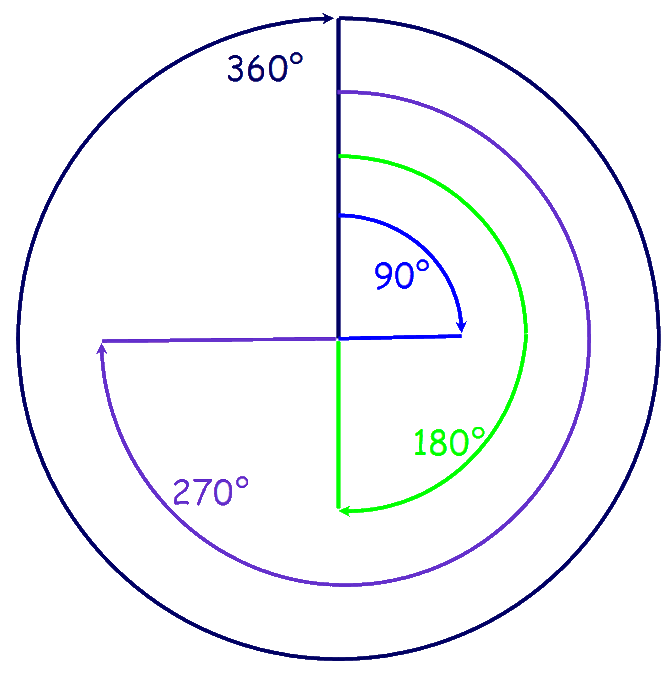## Names of angles

### There are special names for each type of angle:

An angle of 90° is called a 'Right Angle'

An angle less than 90° is called an 'Acute Angle'

An angle more than 90° but less than 180°
is called an 'Obtuse Angle'

An angle more than 180° but less than 360°
is called a 'Reflex Angle'

An angle that equals 180° is a straight line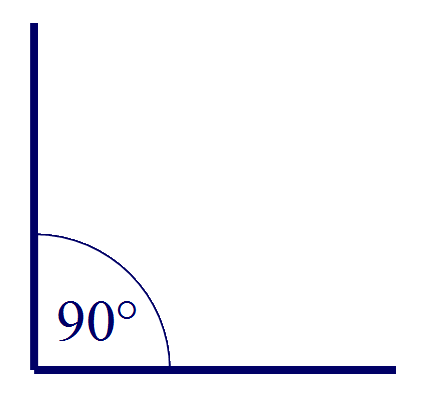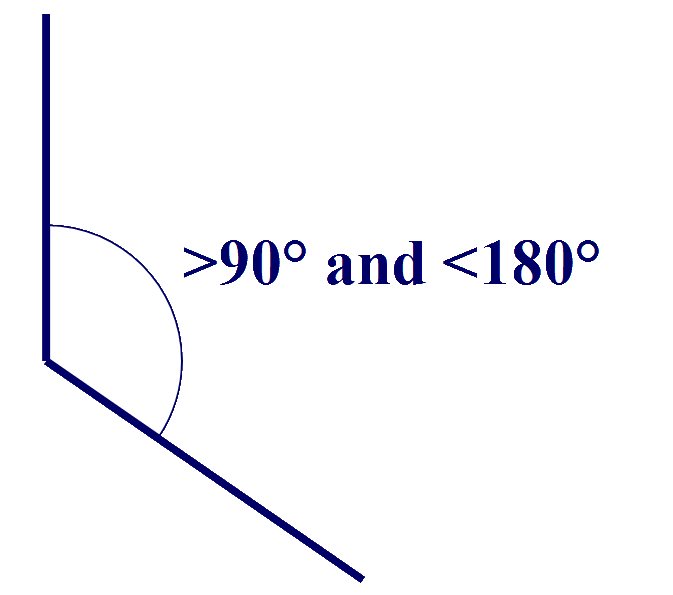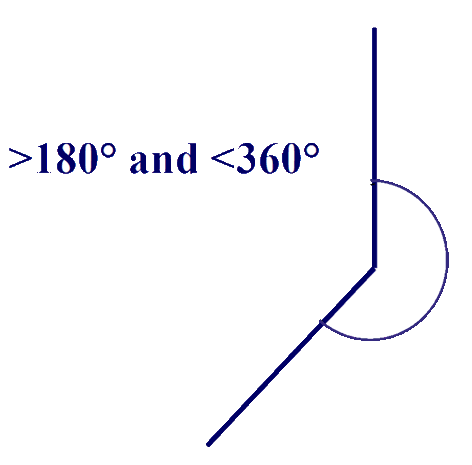### We measure angles with a protractor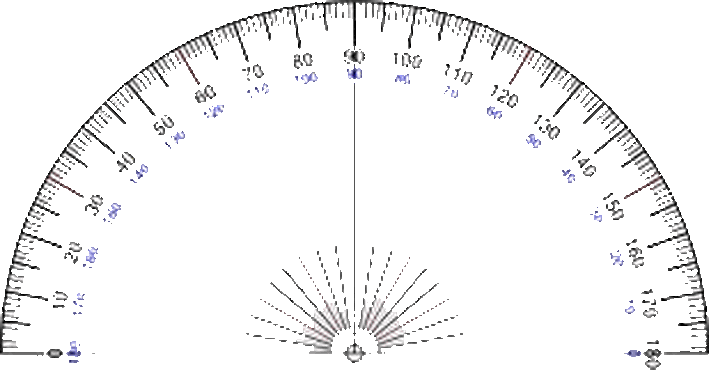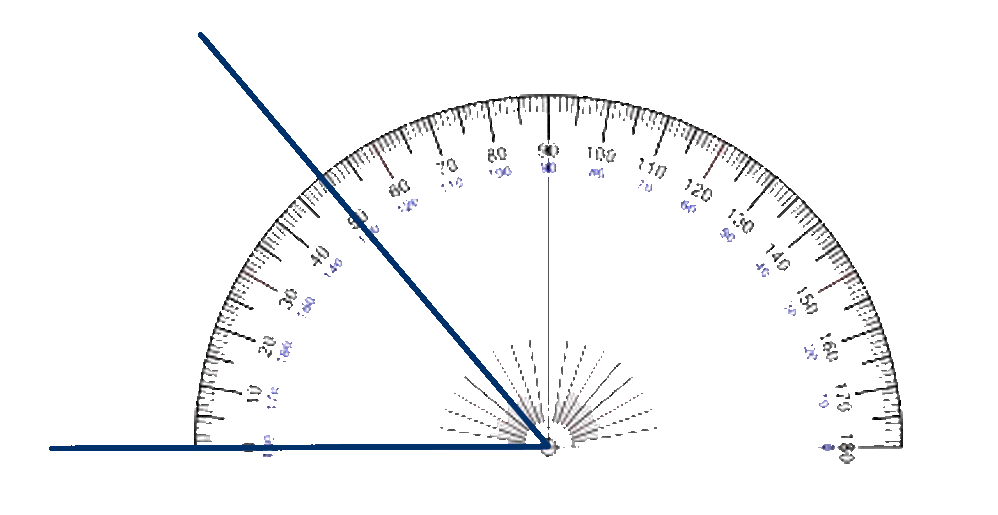To use a protractor, you position the protractor over the angle to be measured with the centre lined up exactly over the point of the angle and the zero line exactly over one line of the angle. Then look at where the other line of the angle intersects with the protractor and read off the angle - in this case 50°

These worksheets from Primary Resources
will help with angles.